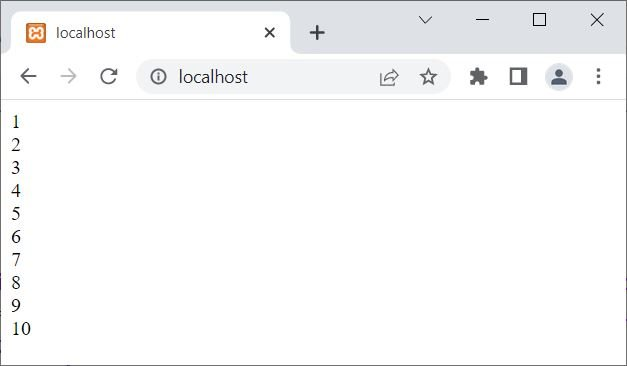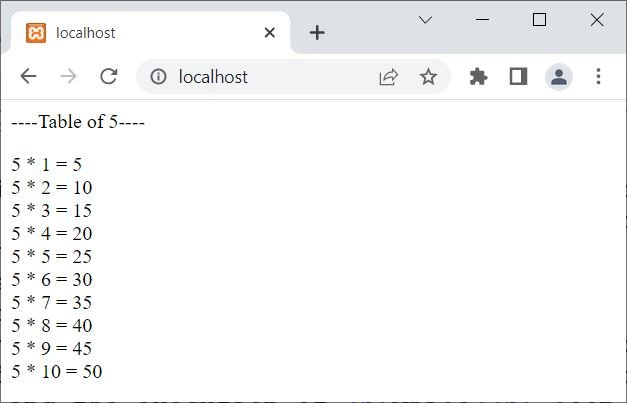# while loop in PHP with examples

The "while" loop in PHP is used when we need to execute a block of code multiple times.

## PHP while loop syntax

The syntax to use the "while" loop in PHP is as follows:

```while(condition)
{
// body of the loop
}```

The "body of the loop" will continue its execution until the "condition" is evaluated to be false. For example:

```<?php
\$x = 1;
while(\$x<=10)
{
echo \$x, "<BR>";
\$x++;
}
?>```

The output produced by the above PHP example is:## PHP while loop example

The following PHP code can be considered an example of the "while" loop.

```<?php
\$num = 2;
while(\$num<=20)
{
echo \$num, "<BR>";
\$num = \$num+2;
}
?>```

The output of the above PHP example is shown in the snapshot given below:Note: Whatever the expression inside of while() is, it will be considered a conditional expression. After evaluating a conditional expression, a boolean value (true or false) gets returned. If the boolean value is true, then the execution of the while loop continues; otherwise, if the conditional expression evaluates to be false, then the execution of the while loop stops.

The dry run for the preceding example is as follows:

1. A variable \$num is defined with its value as 2.
2. Now the execution of the while loop begins.
3. Since at first the condition \$num<=20 or 2<=20 evaluates to be true.
4. Therefore, program flow goes inside the loop.
5. Using the statement:
`echo \$num, "<BR>";`
6. The value of \$num, which is 2, along with a line break, will be printed on the output.
7. Now for the second statement of the loop, that is:
`\$num = \$num+2;`
gets executed
8. Therefore, \$num+2 or 2+2 or 4 will be initialized to \$num. Now \$num = 4.
9. As all the statements inside the loop have been executed, the program flow again goes to the conditional expression of the while loop to check the condition again.
10. The second time, the condition \$num<=20 or 4<=20 evaluates to be true again.
11. Therefore, program flow again goes inside the loop.
12. Now the similar process goes again, from step no. 5 to step no. 9, with of course the new value of \$num.
13. This process continues until the value of \$num becomes greater than 20.
14. Because when the value of \$num becomes greater than 20, the condition \$num<=20 evaluates to be false, and the execution of the while loop stops.

Let me create another example using the while loop in PHP:

```<?php
\$num = 5;
\$i = 1;
echo "<p>----Table of \$num----</p>";
while(\$i<=10)
{
echo "\$num * \$i = ", \$num*\$i, "<BR>";
\$i++;
}
?>```

Now the output should be:Following is one last example of a while loop in PHP:

```<?php
\$sum = 0;
\$count = 0;
\$check = true;

while(\$check)
{
if(\$sum === 500)
\$check = false;
else
\$sum += 10;
\$count++;
}
\$count--;

echo "<p>Value of \\$sum = ", \$sum, "</p>";
echo "<p>Number of times, loop executed = ", \$count, "</p>";
?>```

The output should be:

```Value of \$sum = 500

Number of times, loop executed = 50```

### Advantages of the while loop in PHP

• "while" loops are extremely flexible and can be used for a variety of tasks, including iterating over arrays and performing complex calculations.
• "while" loops can be more efficient than other types of loops, such as "for" loops, when the number of times the loop must execute is unknown in advance.
• "while" loops are simple to comprehend and employ, making them a good option for beginners or simple tasks.

### Disadvantages of the while loop in PHP

• Risk associated with infinite loops: If the condition in a "while" loop is never false, the loop will continue to execute indefinitely, causing your script to crash or hang.
• Sometimes "while" loops can become overly complicated, especially when multiple conditions and nested loops are involved.
• "while" loops do not have built-in control over the number of iterations, so you must manually control the loop exit conditions, which can be difficult for complex tasks.

PHP Online Test

« Previous Tutorial Next Tutorial »